•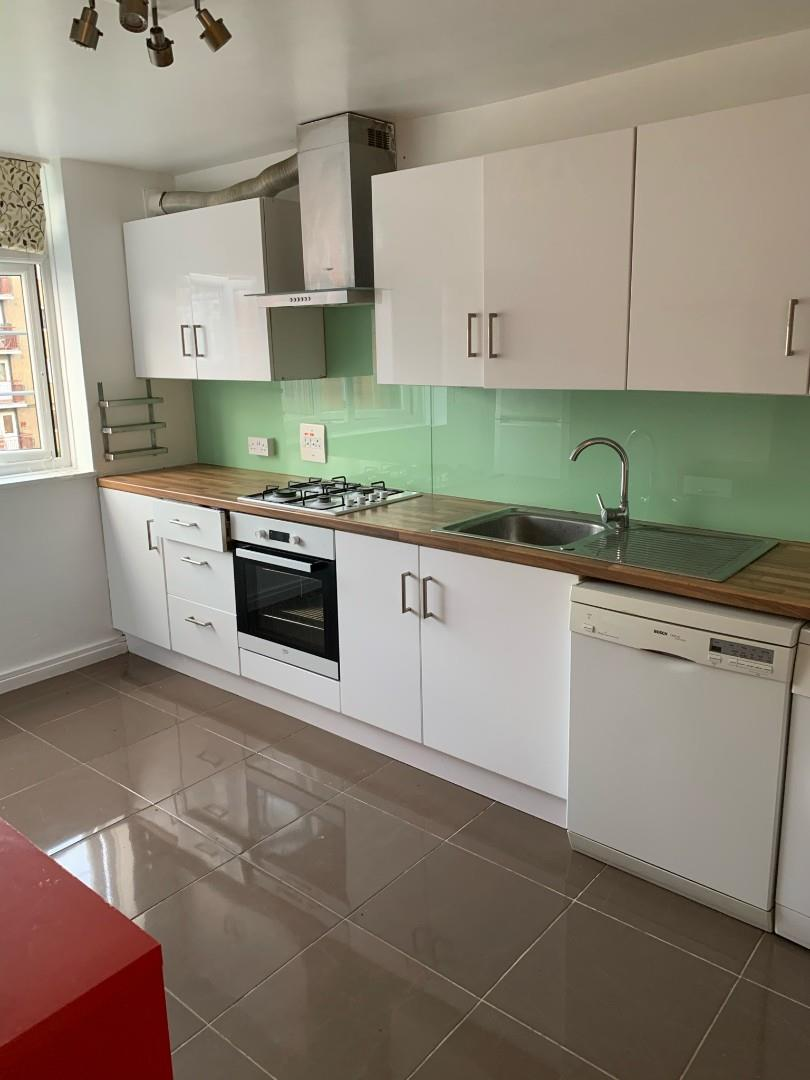£1,500.00 To Let
•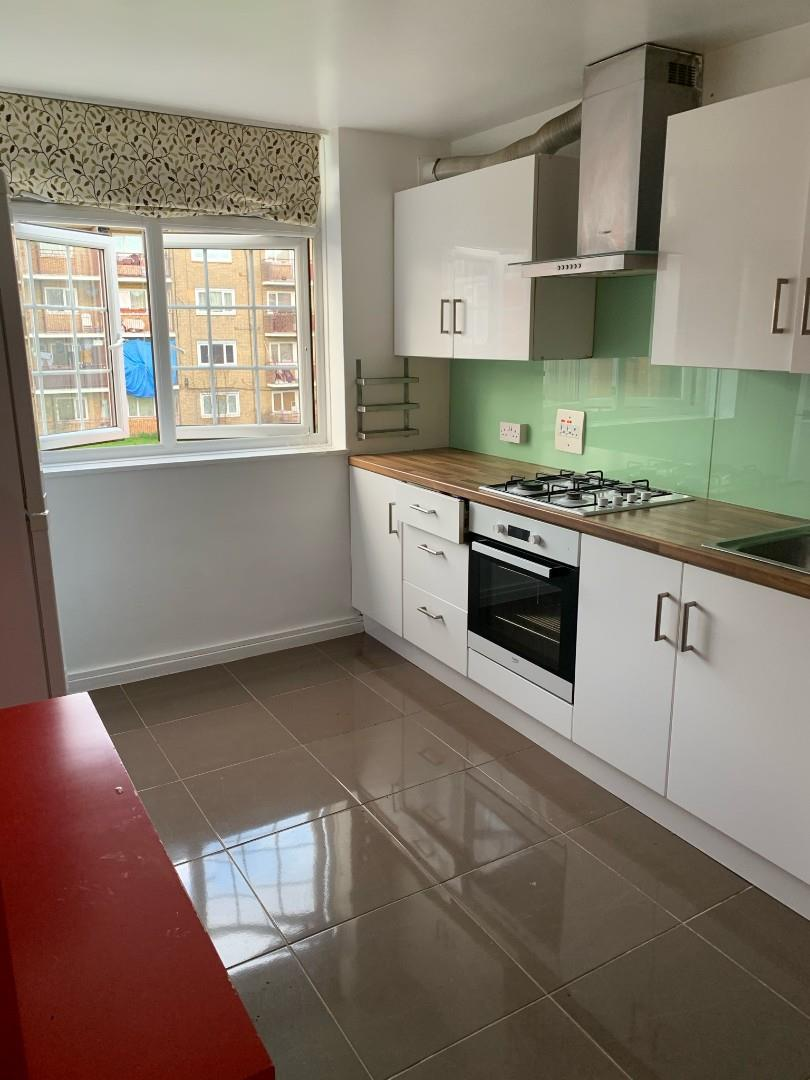£1,500.00 To Let
•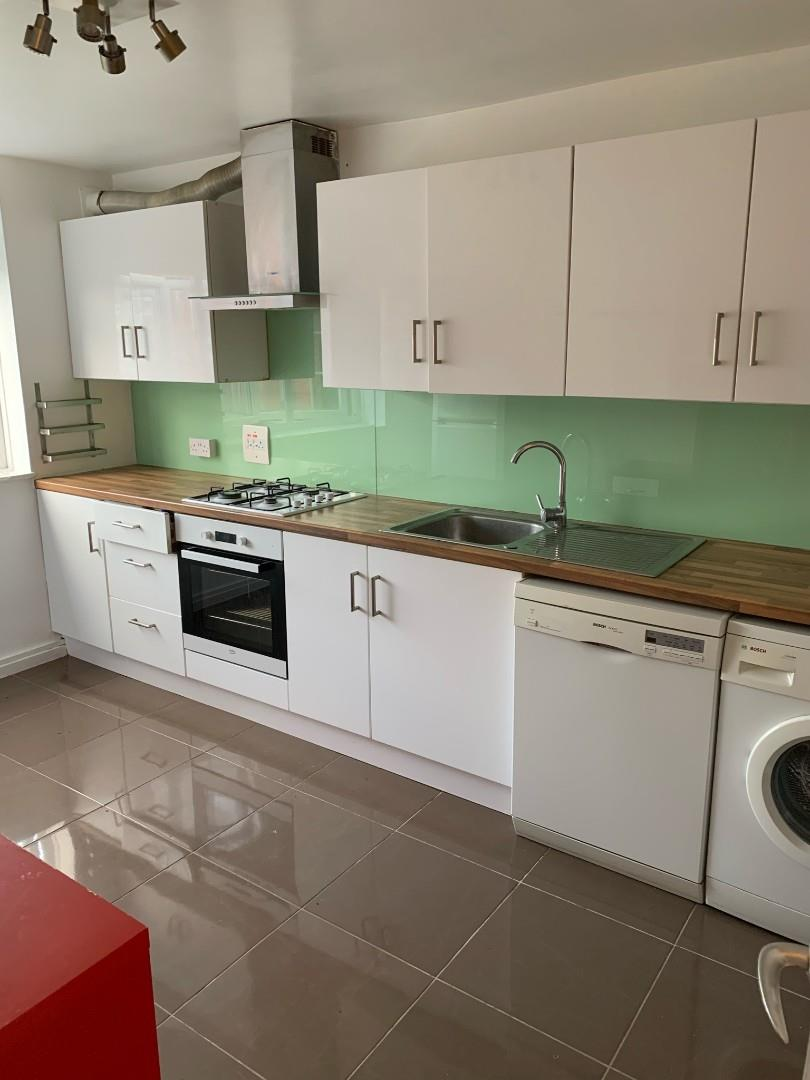£1,500.00 To Let
•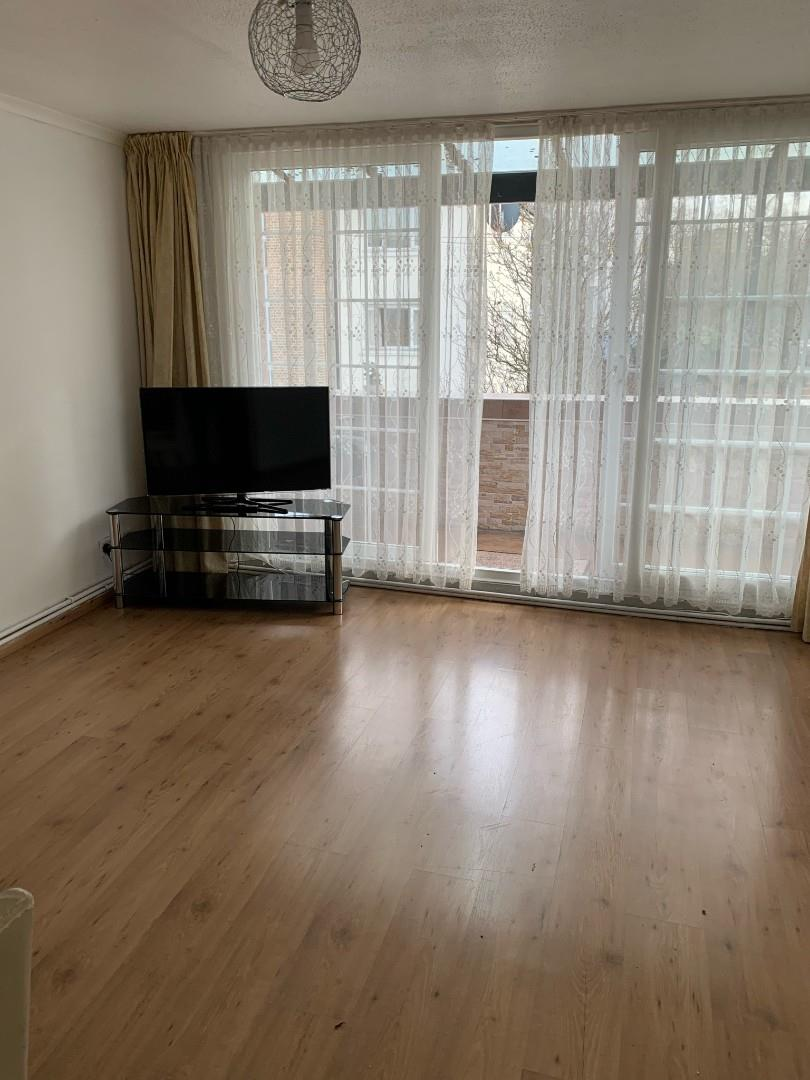£1,500.00 To Let
•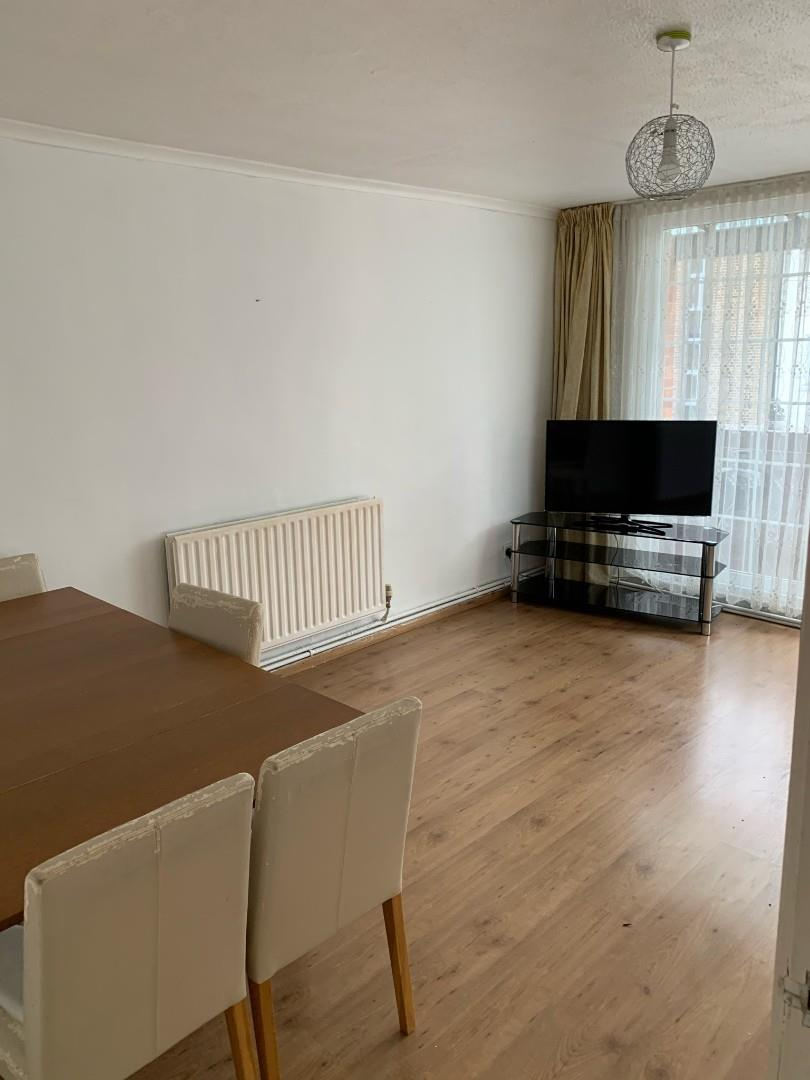£1,500.00 To Let
•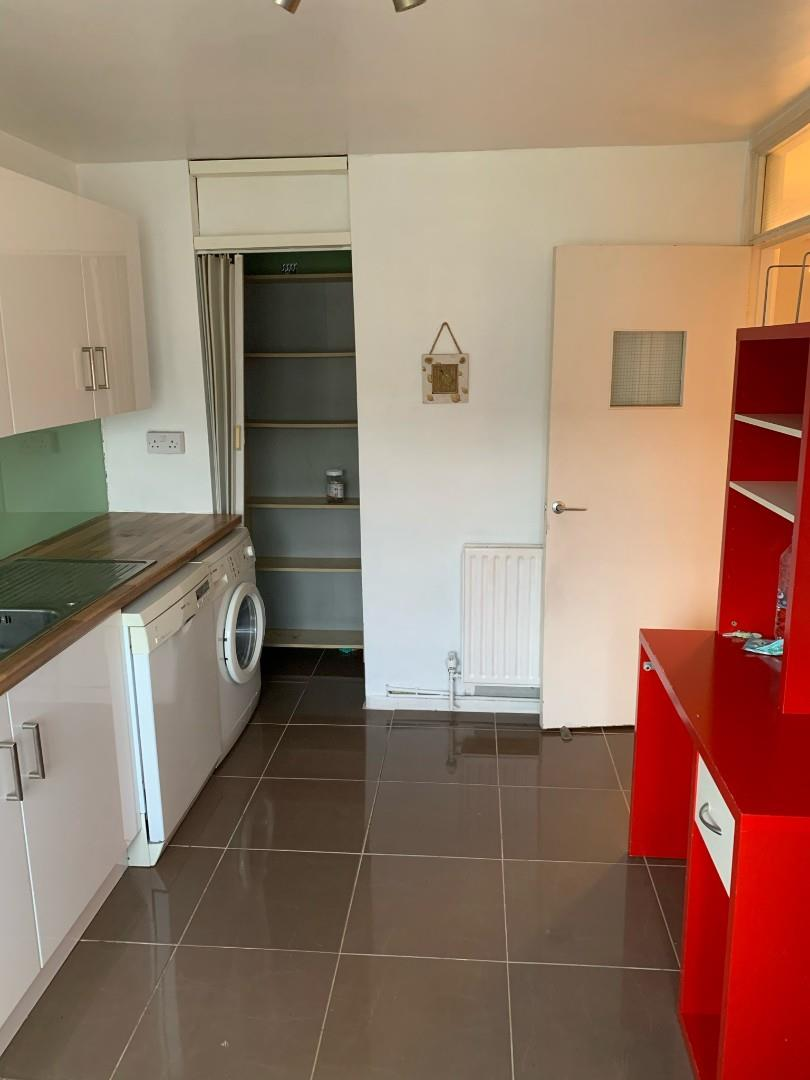£1,500.00 To Let
•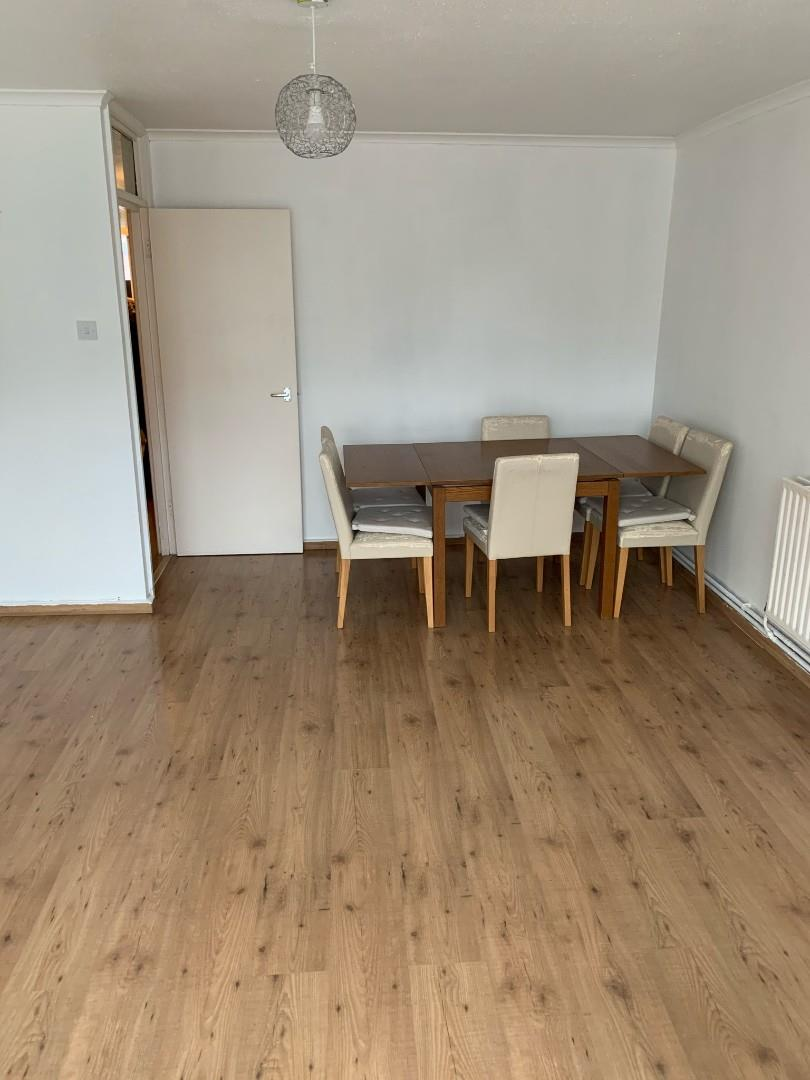£1,500.00 To Let
•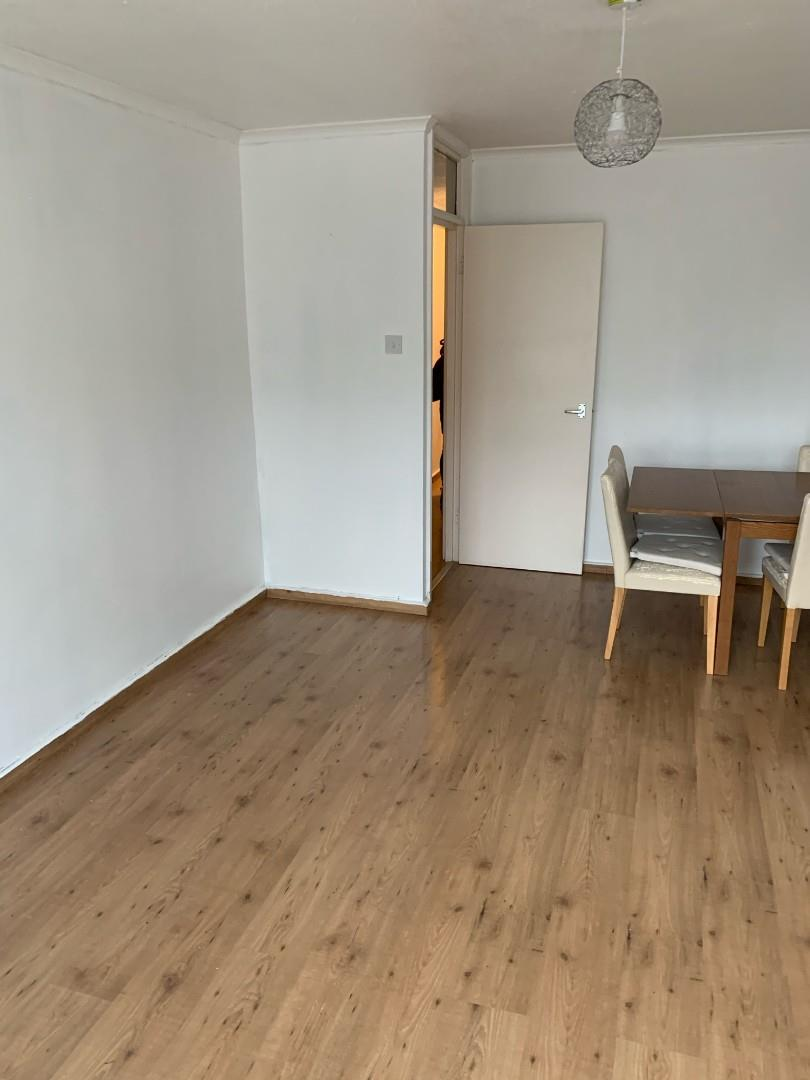£1,500.00 To Let
•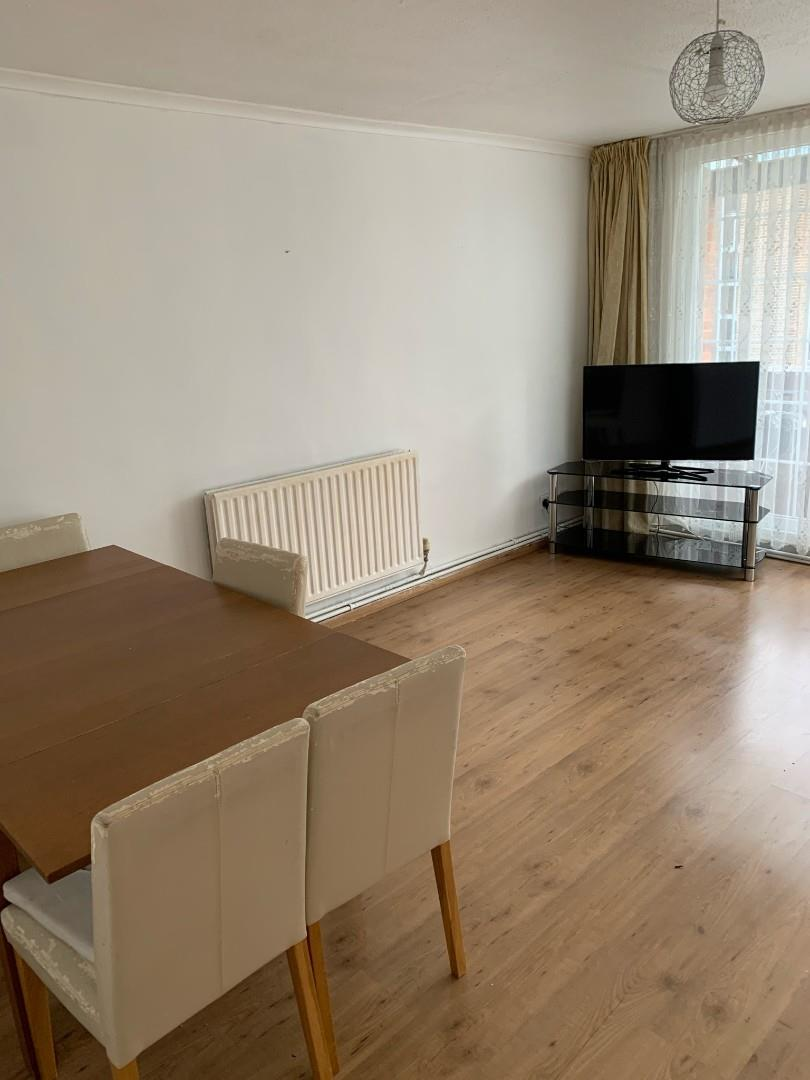£1,500.00 To Let
•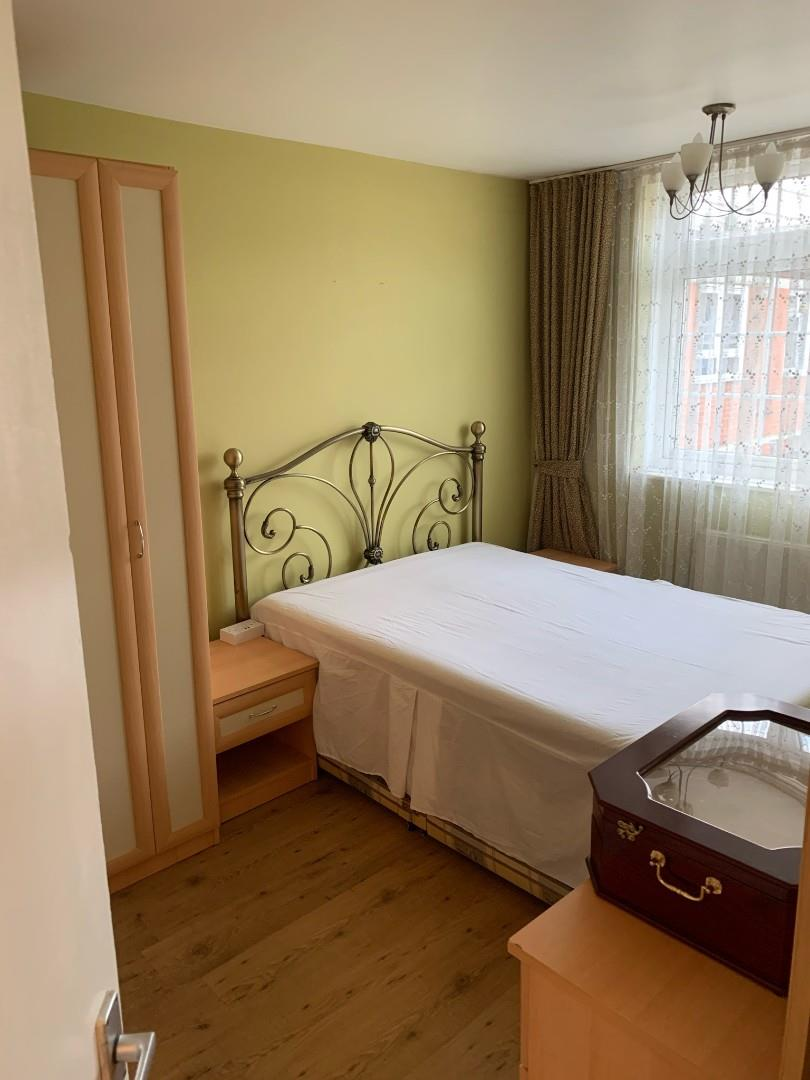£1,500.00 To Let
•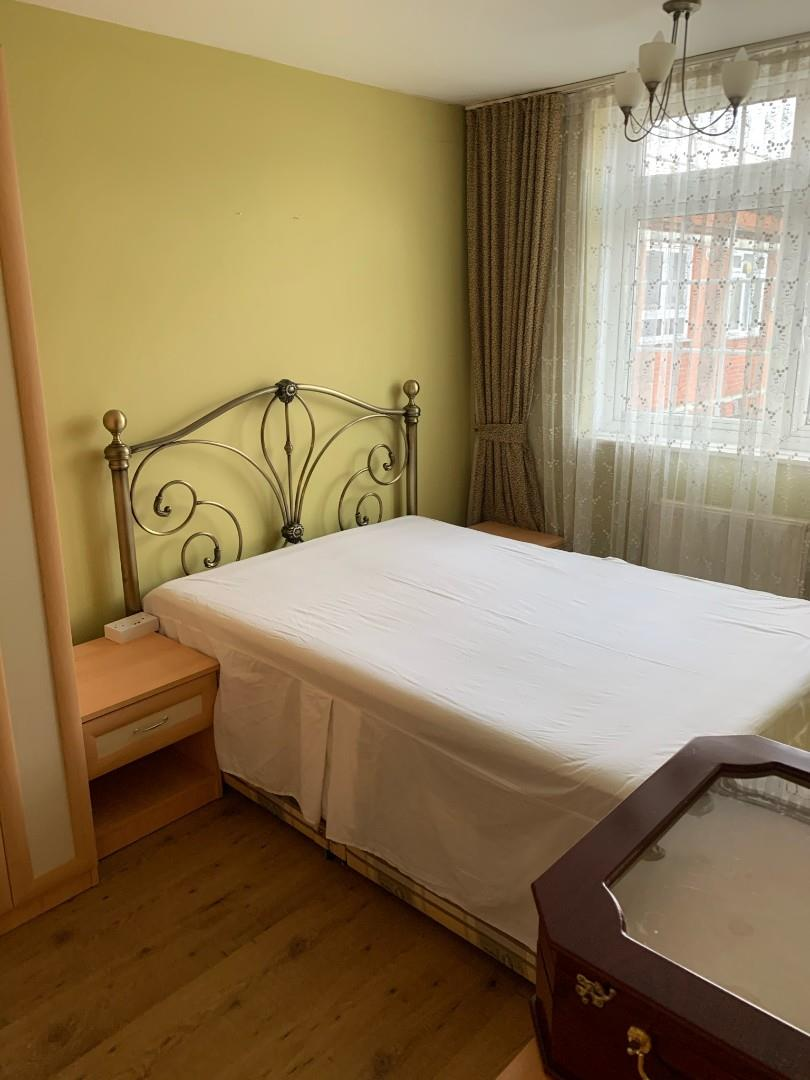£1,500.00 To Let
•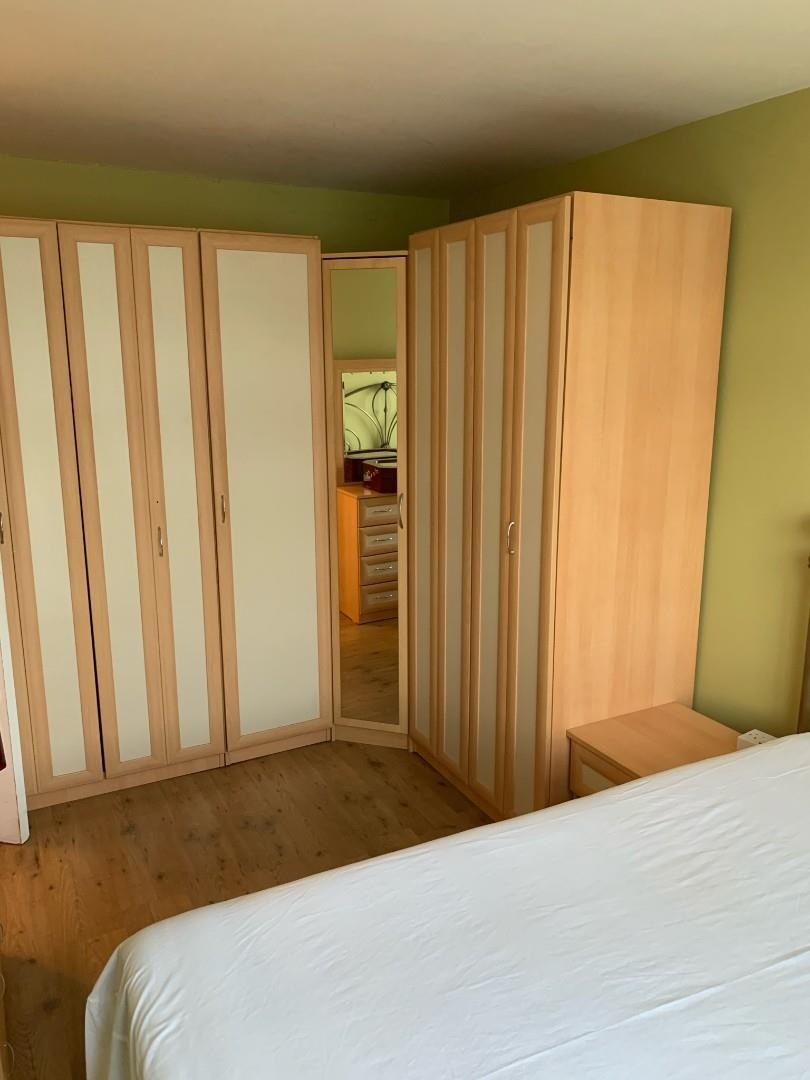£1,500.00 To Let
•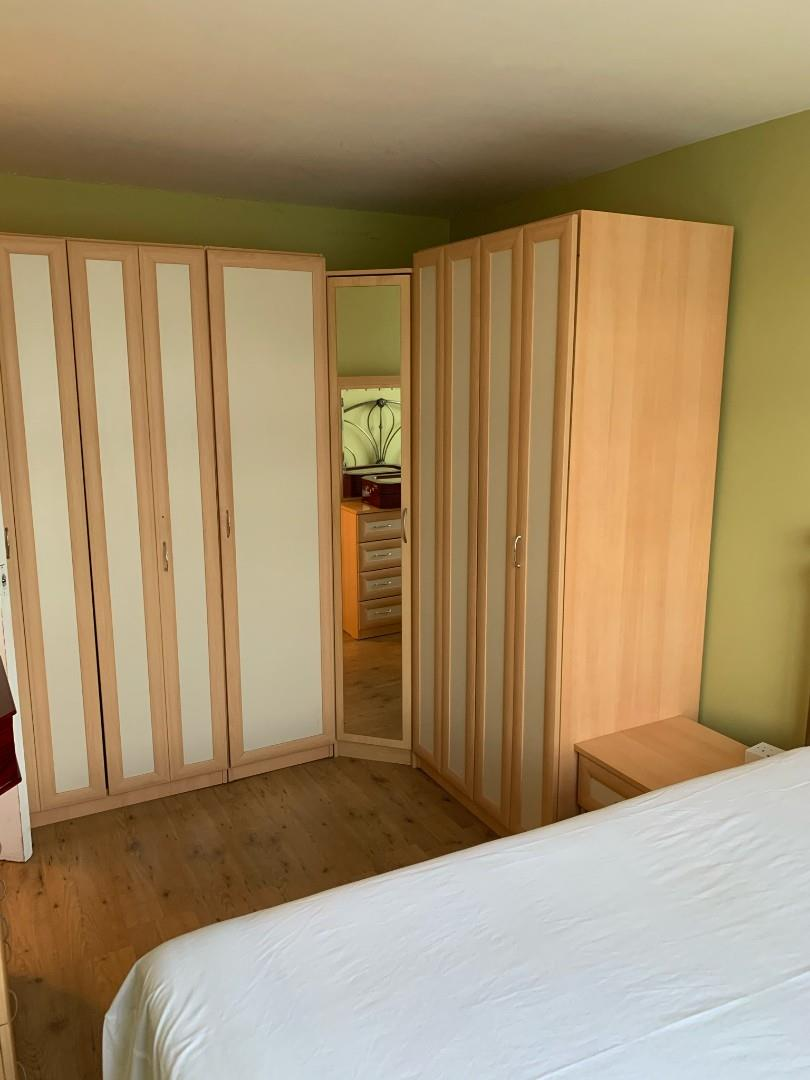£1,500.00 To Let
•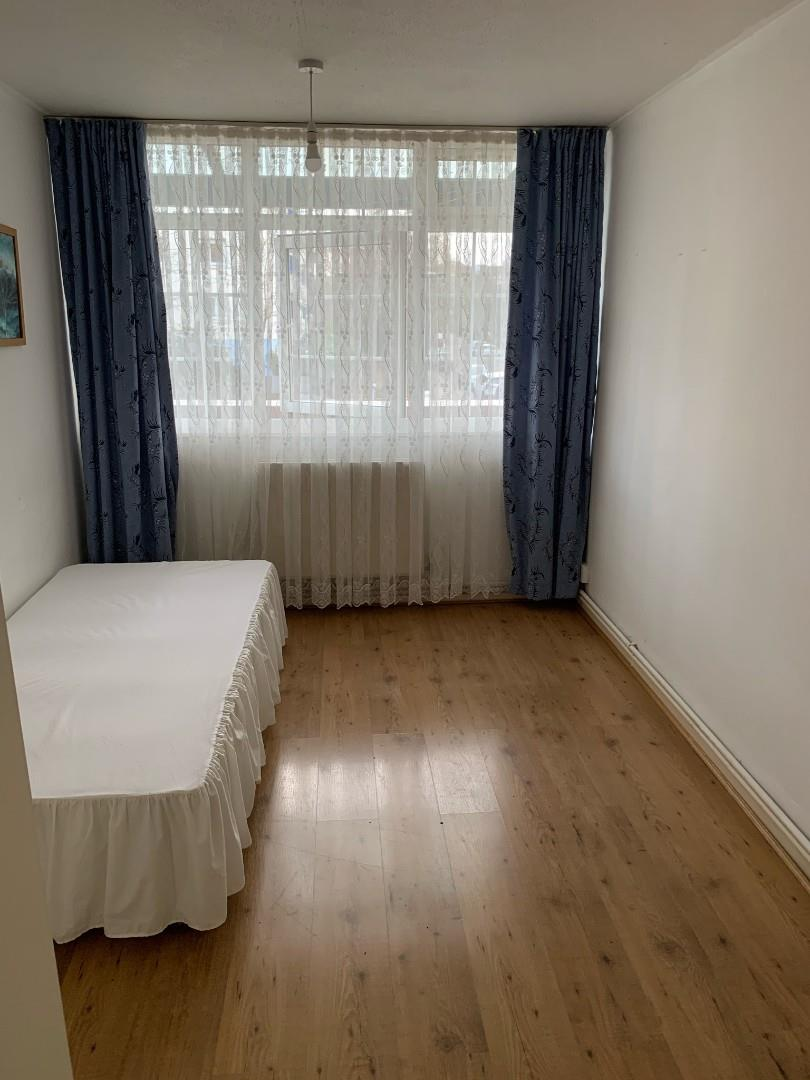£1,500.00 To Let
•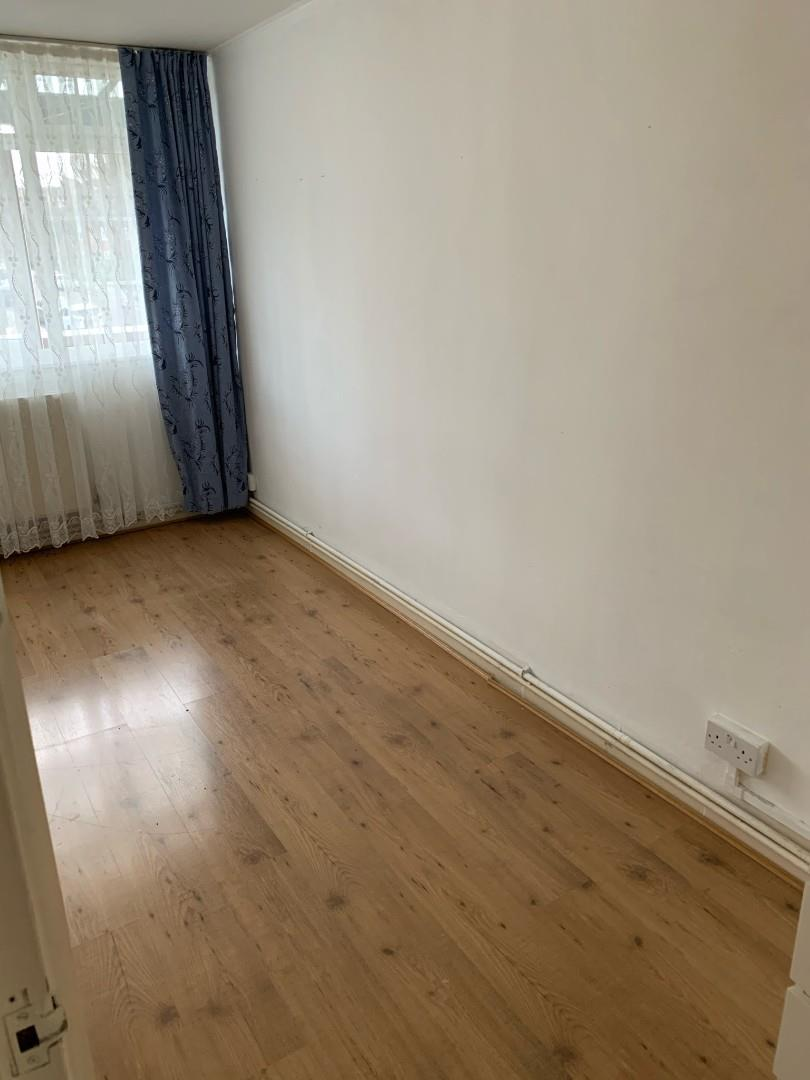£1,500.00 To Let
•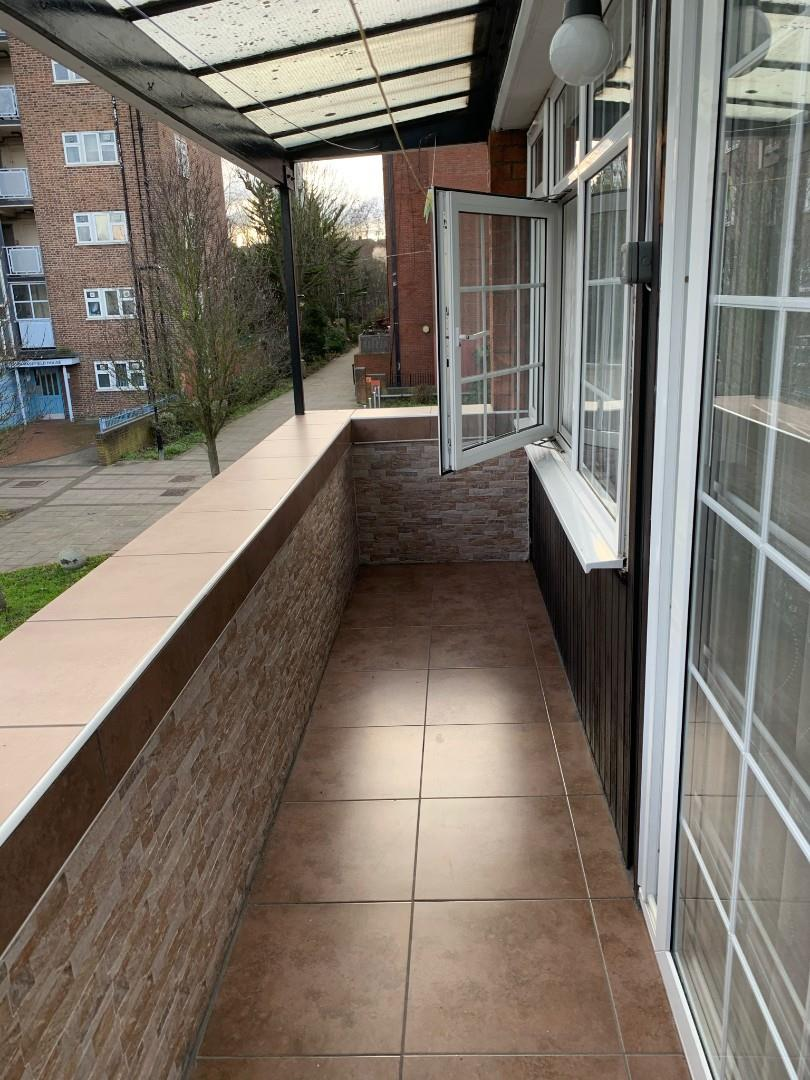£1,500.00 To Let
•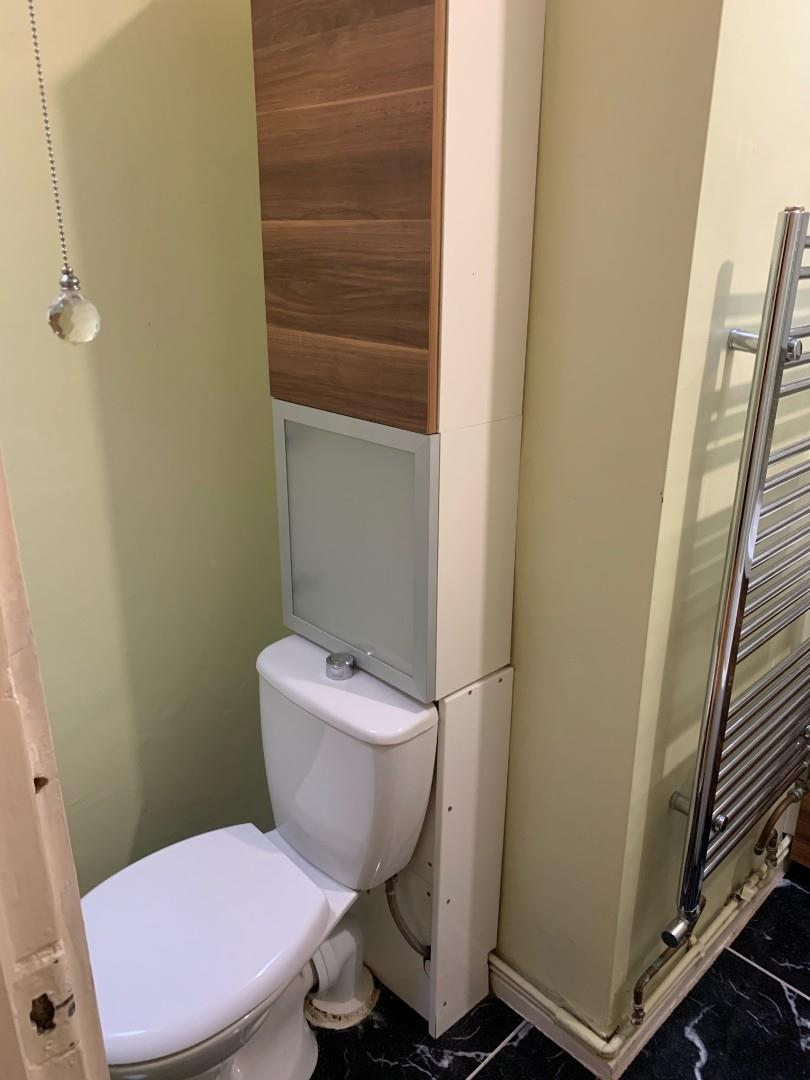£1,500.00 To Let
•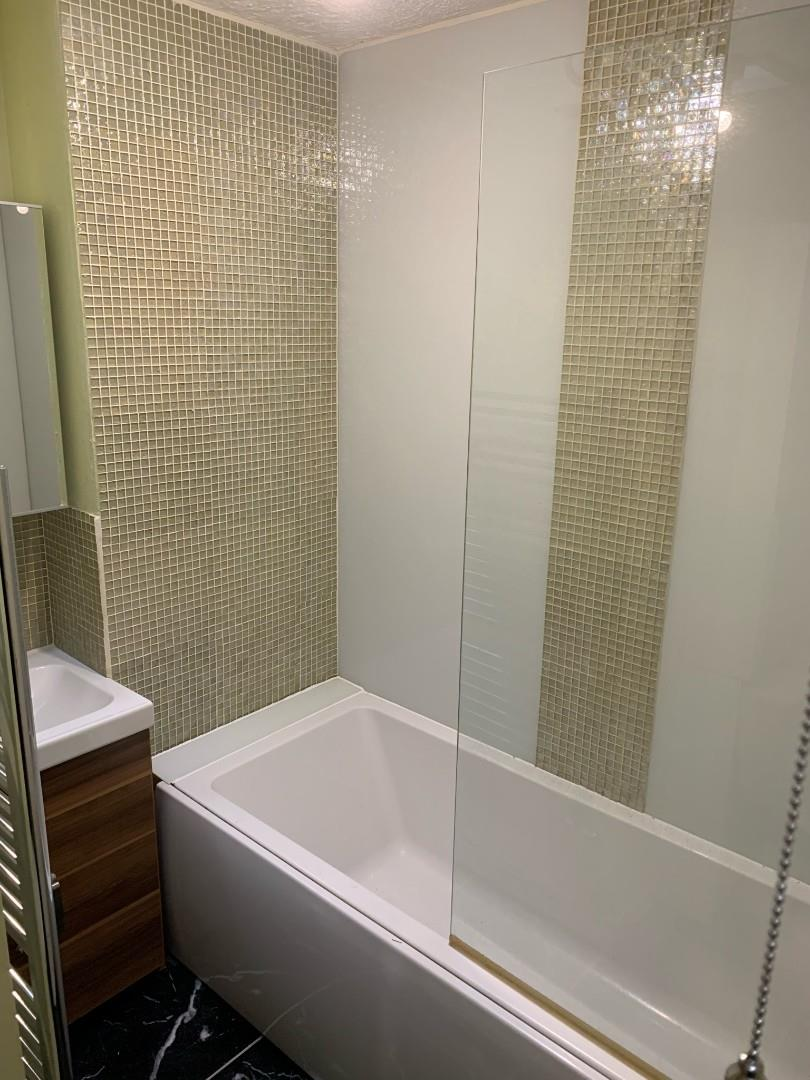£1,500.00 To Let
•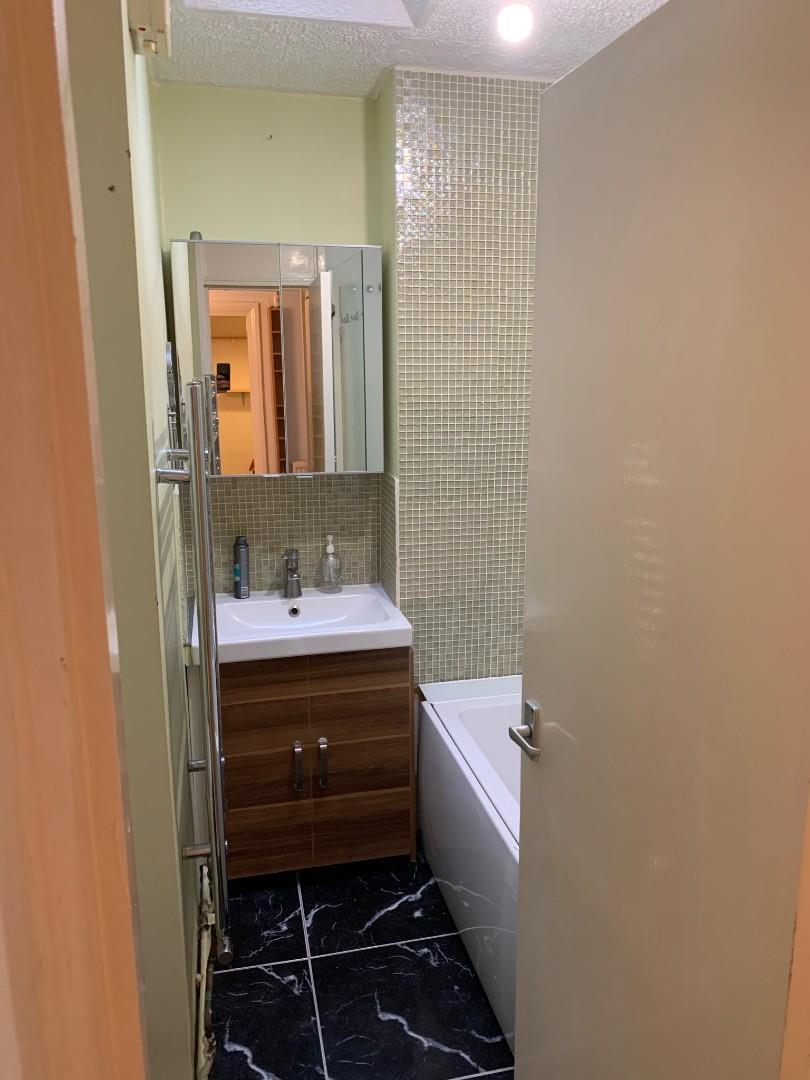£1,500.00 To Let
•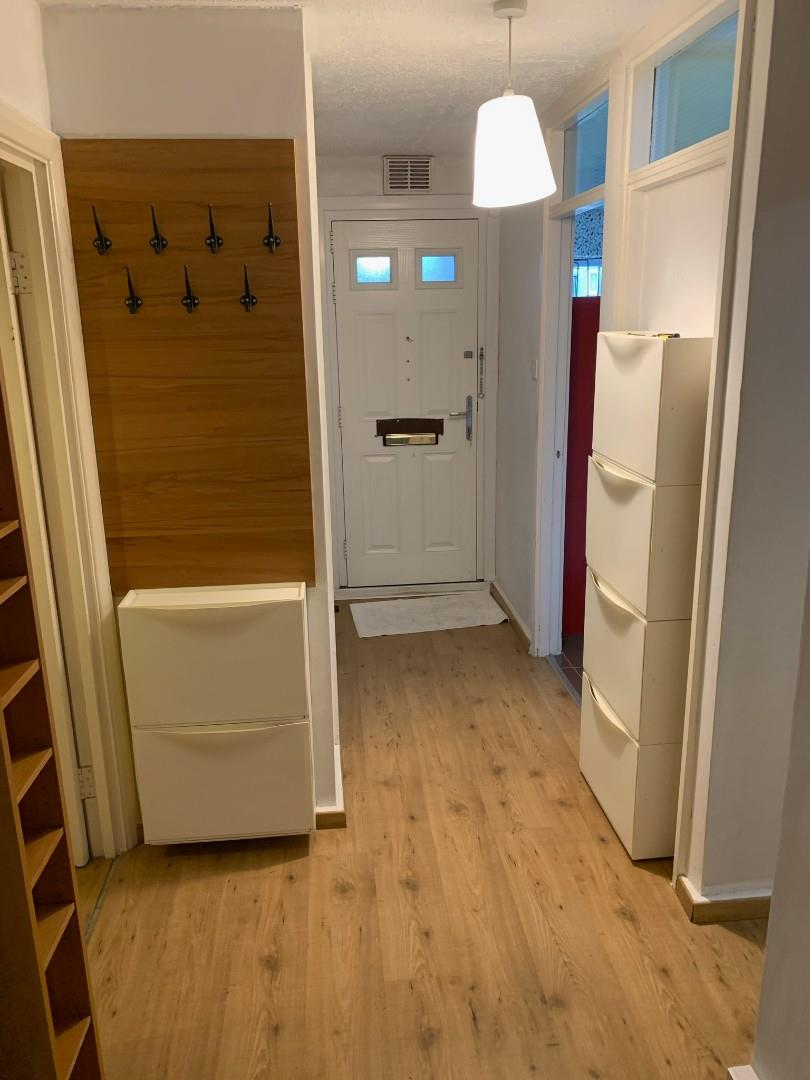£1,500.00 To Let
•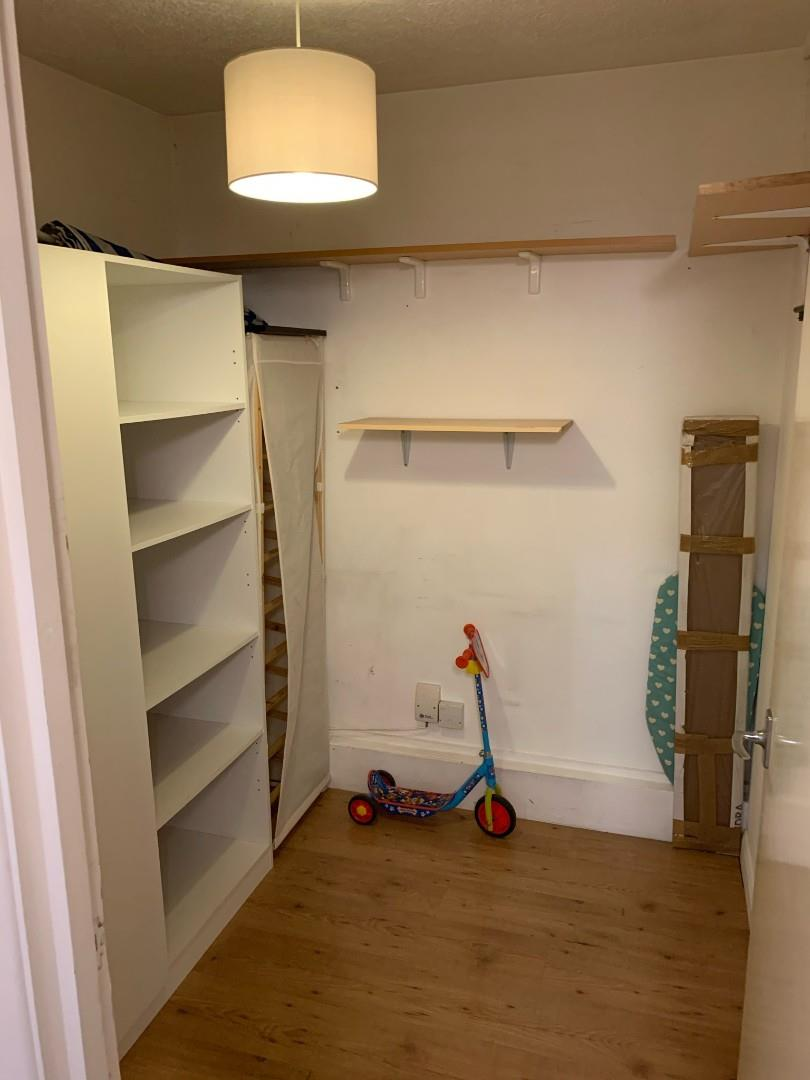£1,500.00 To Let
•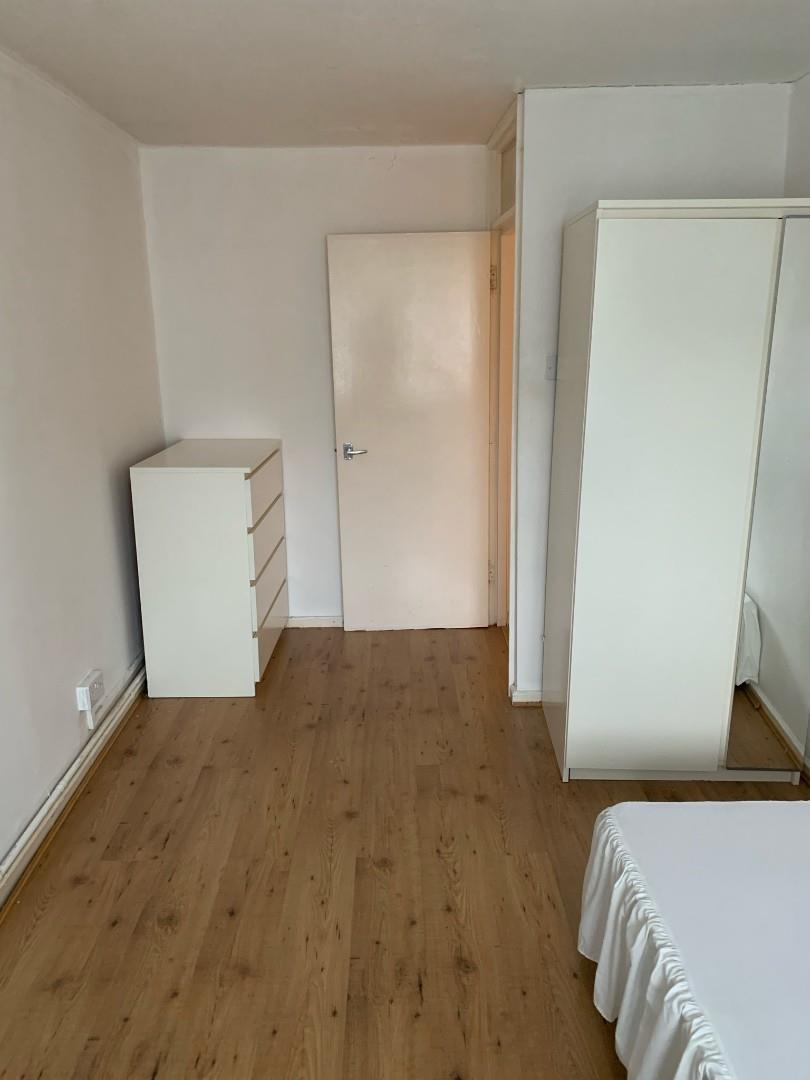£1,500.00 To Let
•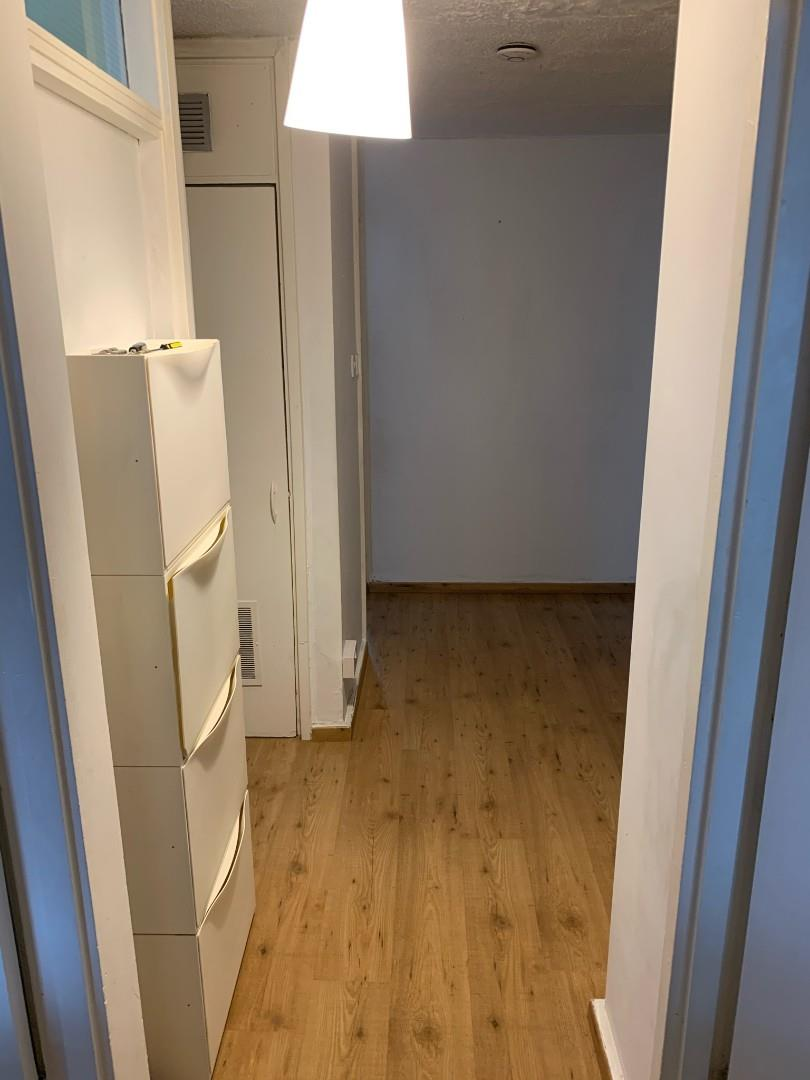£1,500.00 To Let
•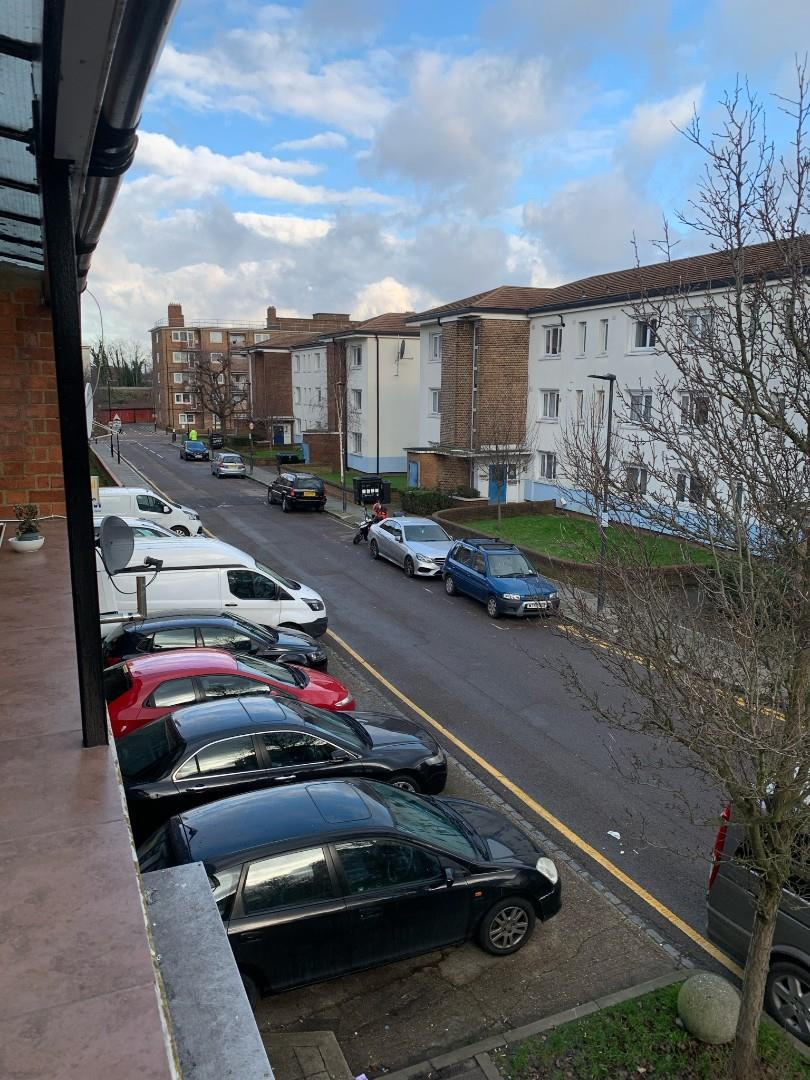£1,500.00 To Let
•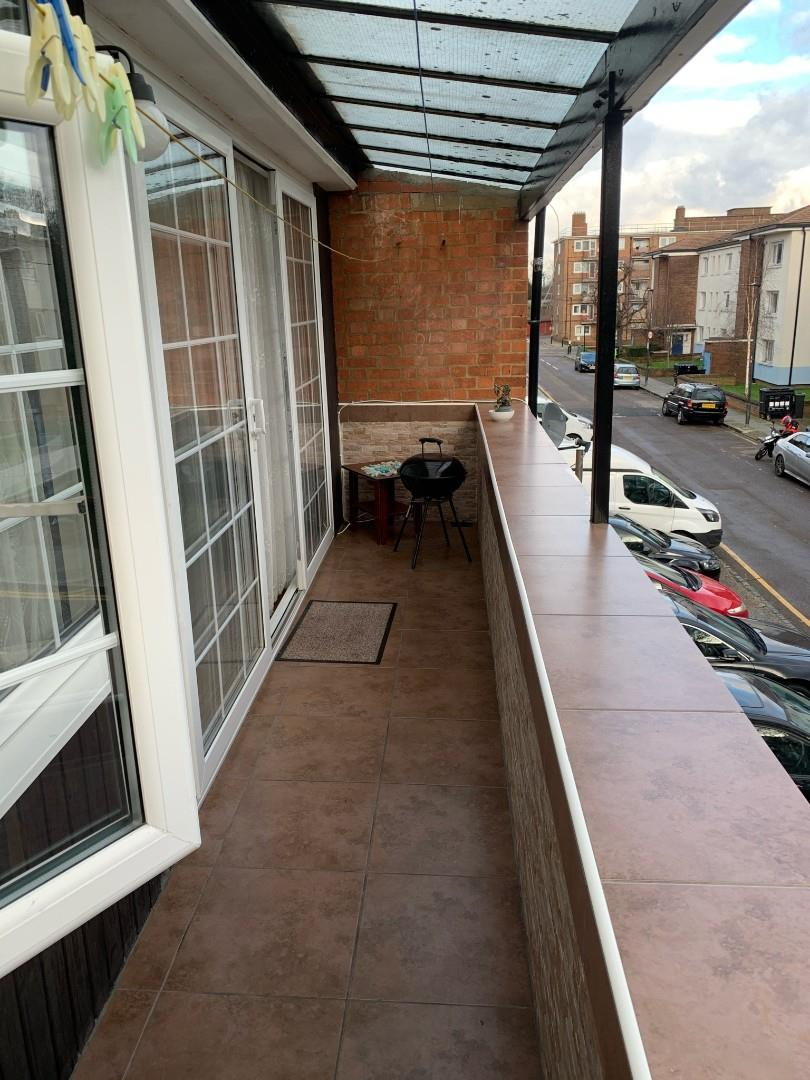£1,500.00 To Let
•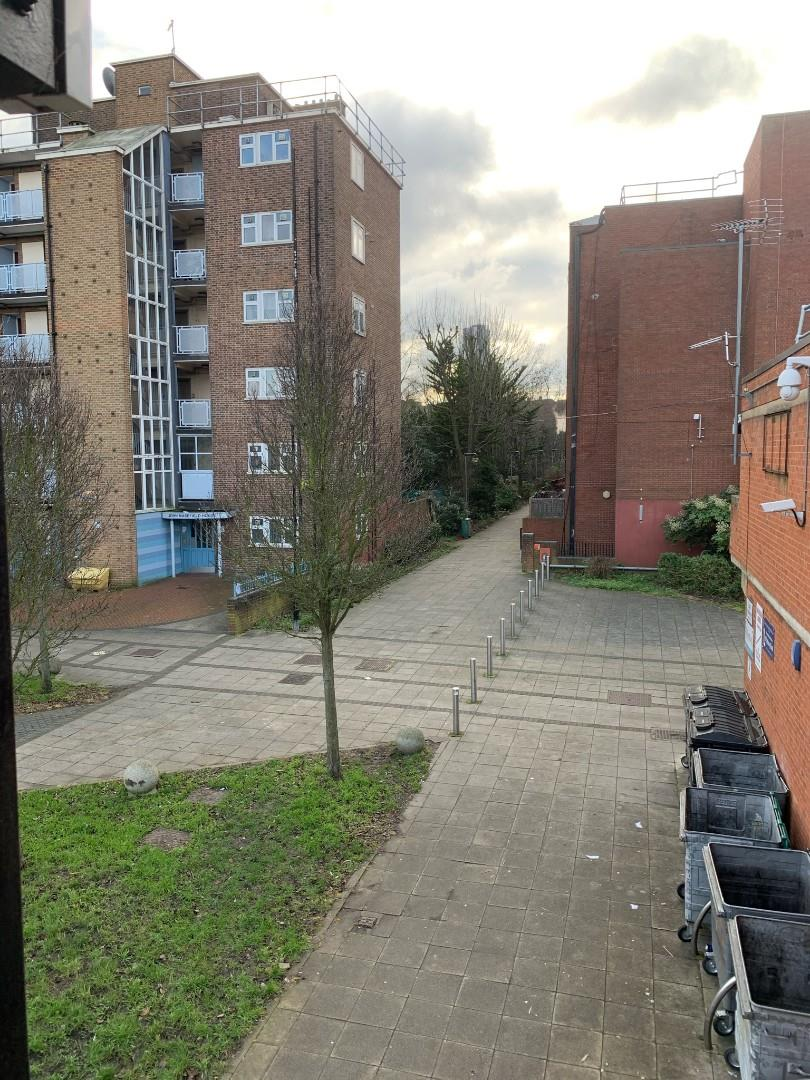£1,500.00 To Let
•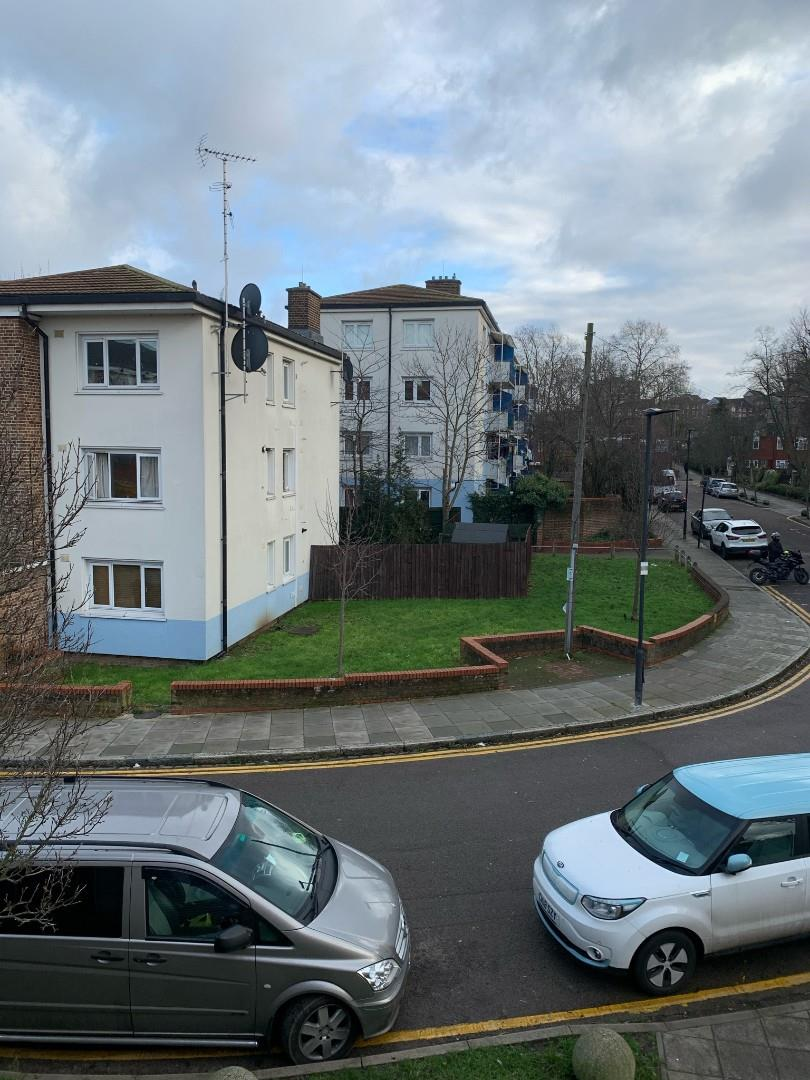£1,500.00 To Let
•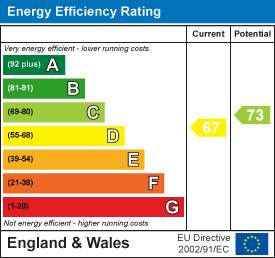£1,500.00 To Let